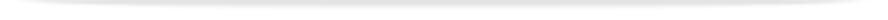units

MEC3457

Faculty of Engineeringprint version

This unit entry is for students who completed this unit in 2016 only. For students planning to study the unit, please refer to the unit indexes in the the current edition of the Handbook. If you have any queries contact the managing faculty for your course or area of study.

MEC3457 - Systems and control

6 points, SCA Band 2, 0.125 EFTSL

Refer to the specific census and withdrawal dates for the semester(s) in which this unit is offered.

Faculty

Engineering

Organisational Unit

Department of Mechanical and Aerospace Engineering

Coordinator(s)

Prof Sunita Chauhan (Clayton); Dr Wang Xin (Malaysia)

Offered

Clayton

• Second semester 2016 (Day)

Malaysia

• Second semester 2016 (Day)

Synopsis

This unit covers the nature and behaviour of simple components, processes and subsystems relevant to engineering control. Mechanical, electrical, fluid pressure devices and complete elementary control systems are included. Orientation is to predicting, examining and assessing system performance via formation of mathematical models and solution of models. Laboratory experiments and hands-on instruction in the digital simulation package Matlab to solve models. A unified approach to mathematical modelling via the concepts of resistance, capacitance and inertia/inductance is emphasised. Students learn to perform system modelling, develop solution, assess a system response and analyse systems.

Outcomes

• Understand the significance and relevance of systems and associated control in engineering.
• Appreciation for mathematical formulations to develop accurate linear models through classical and state space modelling techniques describing systems with single-input single-output (SISO) and multi-input and multi-output (MIMO).
• Gain knowledge of system's response through the use of analytical techniques, such as classical solution, Laplace transforms, state space, etc.
• Understand the concept of stability and its importance for systems analysis and control.

Gain knowledge of dynamic performance of a system, including selection of system parameters to achieve the desired response, and further analysis through techniques such as frequency response.

• Understand the effects of non-linearity in systems and limitations of the use of linear models.
• Gain knowledge of experimental, as well as computer-based (such as Matlab) techniques.
• Ability to develop mathematical models for devices and systems for the purpose of response and dynamic behaviour analysis.
• Ability to obtain system's solution and response using classical method, Laplace transforms method, and state space method.
• Ability to determine the stability of a system utilising S-plane and Routh-Hurwitz technique.
• Ability to analyse dynamic performance of systems in the time and frequency domains using the Bode plot, root-locus, and Nyquist plot.
• Ability to continue learning about systems modelling and control techniques beyond the content provided in this course.
• An appreciation of the unified concept of resistance, capacitance and inertia/indusctance to perform physical modelling of mechanical, fluidic, hydraulic and pneumatic systems.
• An appreciation for the need to represent, predict and analyse the system and its response and dynamic performance, and convey these through pictorial representations such as block diagrams, signal flow graphs, plots, etc
• An appreciation of non-linear effects and the use of linear models as approximation.

Assessment

Continuous assessment 50%
Examination (3 hours): 50%

Students are required to achieve at least 45% in the total continuous assessment component and at least 45% in the final examination component and an overall mark of 50% to achieve a pass grade in the unit. Students failing to achieve this requirement will be given a maximum of 45% in the unit.

3 hour lectures, 3 hours practice sessions or laboratories (this may alternate with 2 hours lectures and 4 hours practice sessions/laboratories depending on the week) and 6 hours of private study per week